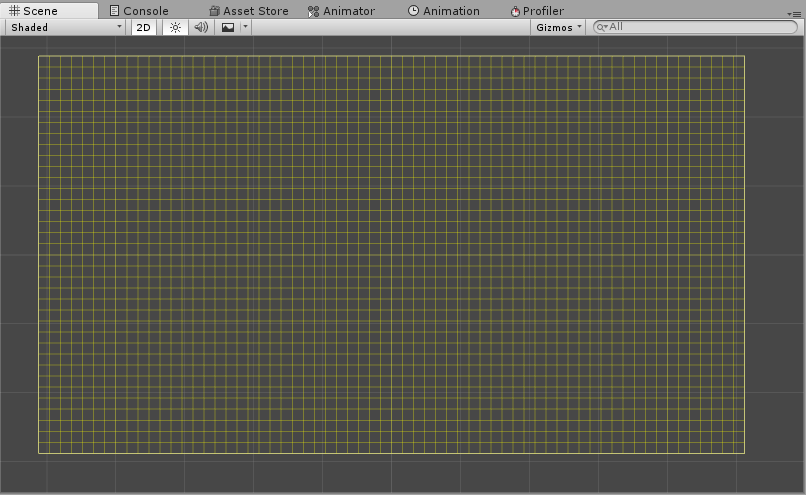# ソースコード

```using UnityEditor;
using UnityEngine;

public static class SceneViewCustom
{
private static readonly int     SIZE        = 16;
private static readonly int     SIZE_HALF   = SIZE / 2;
private static readonly int     WIDTH       = 1024;
private static readonly int     WIDTH_HALF  = WIDTH / 2;
private static readonly int     HEIGHT      = 576;
private static readonly int     HEIGHT_HALF = HEIGHT / 2;
private static readonly int     COLUMN      = WIDTH / SIZE;
private static readonly int     ROW         = HEIGHT / SIZE;
private static readonly Color   COLOR       = new Color32( 255, 255, 0, 100 );

static SceneViewCustom()
{
SceneView.onSceneGUIDelegate += OnSceneGUIDelegate;
}

private static void OnSceneGUIDelegate( SceneView sceneView )
{
Handles.color = COLOR;

for ( int x = 0; x <= COLUMN; x++ )
{
var px = x * SIZE - WIDTH_HALF;
var p1 = new Vector3( px, -HEIGHT_HALF );
var p2 = new Vector3( px,  HEIGHT_HALF );
Handles.DrawLine( p1, p2 );
}

for ( int y = 0; y <= ROW; y++ )
{
var py = y * SIZE - HEIGHT_HALF;
var p1 = new Vector3( -WIDTH_HALF, py );
var p2 = new Vector3(  WIDTH_HALF, py );
Handles.DrawLine( p1, p2 );
}
}
}
```